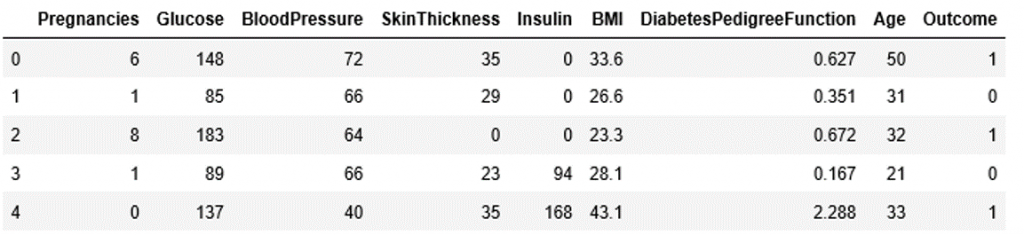### Bagging Technique in Machine Learning

• date 15th February, 2020 |
• by Prwatech |

# Bagging Technique in Machine Learning

Bagging Technique in Machine Learning, in this Tutorial one, can learn Bagging algorithm introduction. Are you the one who is looking for the best platform which provides information about types of bagging algorithms? Or the one who is looking forward to taking the advanced Data Science Certification Course from India’s Leading Data Science Training institute? Then you’ve landed on the Right Path.

Bagging is a powerful ensemble method that helps to reduce variance, and by extension, prevent overfitting. Ensemble methods improve model precision by using a group of models which, when combined, outperform individual models when used separately. The bagging algorithm builds N trees in parallel with N randomly generated datasets with replacement to train the models, the final result is the average or the top-rated of all results obtained on the trees. The Below mentioned Tutorial will help to Understand the detailed information about bagging techniques in machine learning, so Just Follow All the Tutorials of India’s Leading Best Data Science Training institute in Bangalore and Be a Pro Data Scientist or Machine Learning Engineer.

## Bagging algorithm Introduction

As seen in the introduction part of ensemble methods, bagging I one of the advanced ensemble methods which improve overall performance by sampling random samples with replacement. Here it uses subsets (bags) of original datasets to get a fair idea of the overall distribution. Bagging techniques are also called as Bootstrap Aggregation. Generally, these are used in regression as well as classification problems.

### Types of bagging Algorithms

There are mainly two types of bagging techniques.

Bagging meta-estimator

Random Forest

Let’s see more about these types.

### Bagging Meta- Estimator:

It is meta- estimator which can be utilized for predictions in classification and regression problems by means of BaggingClassifier and BaggingRegressor, which are available in scikit learning library. This method involves the following steps:

The original data set is used to create some random subsets which is nothing but bagging or Bootstrapping.

All features are included in the subsets of the data set.

A suitable base estimator is fitted on each of the small sets.

The final result will be a combination of predictions from each mode.

### Random Forest:

It is the most popular technique in the bagging methods category. It is used for classification as well as regression problems. Random forest is nothing but a combination of decisions to identify and locate the data point, inappropriate class. It selects a set of features, only those can decide best split at each node of the decision tree. It works as follows:

Like a bagging meta- estimator, some random subsets are generated from the original dataset.

Only a random set of features are considered to decide the best split, at each node in the decision tree.

Each subset is fitted on the decision tree model.

The final prediction is nothing but the average of the predictions from all decision trees.

Thus, both techniques work to decrease variance and to improve the overall performance of the prediction model rather than a single model.

### Bagging Algorithm Example

To see the working of these techniques, let’s take an example of diabetes prediction. We will consider a common dataset for both techniques. We will divide the main data set into training and testing datasets and will apply each method.

Initializing and importing libraries

import pandas as pd

import numpy as np### Splitting dataset into train and test

from sklearn.model_selection import train_test_split

train, test = train_test_split(df, test_size=0.3, random_state=0)

x_train=train.drop(‘Outcome’,axis=1)

y_train=train[‘Outcome’]

x_test=test.drop(‘Outcome’,axis=1)

y_test=test[‘Outcome’]

### With Bagging Meta- Estimator:

Till this step we separated the training and testing datasets from original set.Now we will apply Bagging Meta- Estimator.As it is classification problem so we will use Baggingclassifier

from sklearn.ensemble import BaggingClassifier

from sklearn import tree

model = BaggingClassifier(tree.DecisionTreeClassifier(random_state=1))

model.fit(x_train, y_train)

model.score(x_test,y_test)

### Output:

0.7619047619047619

Note: In the case of regression, the steps will be the same. Just we have to replace BaggingClassifier with BaggingRegressor.

### With Random Forest:

On the same dataset, we will apply a random forest as follows.

from sklearn.ensemble import RandomForestClassifier

from sklearn.metrics import accuracy_score

model = RandomForestClassifier()

# fitting the model with the training data

model.fit (x_train, y_train)

# fitting the model with the training data

model.fit (x_train, y_train)

pred_train = model.predict(x_train)

# Calculating accuracy on train dataset

Acc_train = accuracy_score(y_train,pred_train)

print(‘\naccuracy_scorefor train dataset : ‘, Acc_train)

### Output:

0.968268156424581

# predicting the target on the test dataset

pred_test = model.predict(x_test)

print(‘\nTarget on test data’,pred_test)

# Calculating accuracy Score for the test dataset

Acc_test = accuracy_score(y_test,predict_test)

print(‘\naccuracy_score on test dataset : ‘, Acc_test)

### Output:

0.8245887445887446

Also, if we want to check the number of decision trees used in building this model, we can just write it as:

print (‘Number of Trees used in the model : ‘, model.n_estimators)

Note: In the case of regression, the steps will be the same. Just we have to replace RandomForestClassifier with RandomForestRegressor.

We hope you understand Bagging Technique in Machine Learning for beginners. Get success in your career as a Data Scientist by being a part of the Prwatech, India’s leading Data Science training institute in Bangalore.

### Quick Support